# Odds Vs Probability

Odds and probability are both terms used to describe the likeliness for something to happen. Many of us mix them up, but in fact, they are quite different, which is why we have added this article to our online casino guide section. Without any numbers, the words can mean the same. If something has high odds of happening, then the probability for the same thing to happen is also high. That said when you start adding numbers and values this changes drastically.

Probability is defined as the percentage of desired outcomes against any possible outcomes within set rules. For example, there’s a 50% chance a coin will land on “heads”. Odds which we hear a lot in sports betting, on the other hand, can have a value of 0 to infinity, and represent a ratio for desired and undesired outcomes. This ratio can be given both in favour of an event happening, but also against the same event happening.
Take a coinflip: It’s a 1:1 odds for the outcome to be TAILS, not 50%.

Now how does this translate to odds and probability we use in our gambler life?

## The Roulette ExampleIn this example, we will look at the probability for red. Later we see this is translated into odds.

First off all, some general information on European Roulette.

• In European Roulette, we’ll find a total of 37 numbers.
• We have 18 Red, and 18 Black slots, in addition to the green “0” where the ball can land.

Let’s try to find the probability of RED to appear in the example below.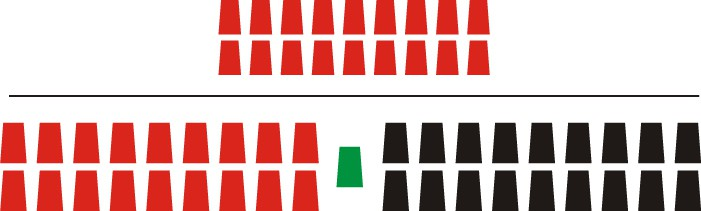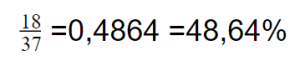You will notice there is no change to the bottom denominator (37). That’s because probability considers the entire roulette table.  Odds, however, find the ratio between favourable and non-favourable outcomes.

## So What are the odds?

Ok, so Let’s look at the odds here. We will use the same event, and odds in FAVOUR for them to happen.

### Odds for Red

First of all, odds look at everything a bit different. Odds compares good outcomes vs bad outcomes, so to write this as fractions, the values are completely different.

Odds in favour of red?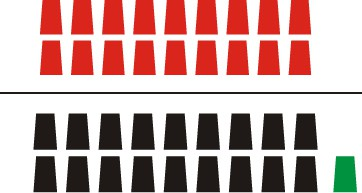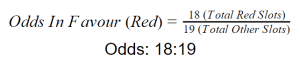And  Odds Against Red?

To find the Odds against the same event, you simply flip the denominators: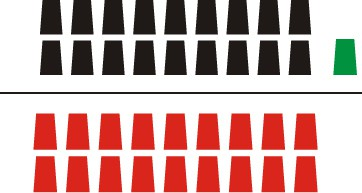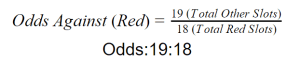We hope you have learned a bit of the basic differences between odds and probability after this quick guide. Want to learn more, and discuss anything gambling? Check out our forums!